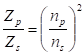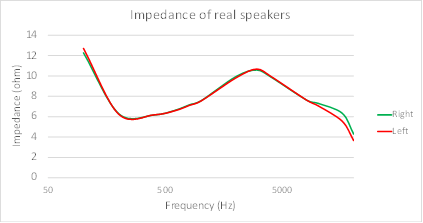The AC impedance of an output transformer, seen by the anode of a vacuum tube when a load (a speaker in our case) is connected to the secondary, depends on the ratio between the number of turns, of the primary and the secondary.

Let np and ns be respectively the number of turns of the primary and secondary. Let Zp, and Zs, be respectively the impedance seen at the primary and the impedance applied to the secondary (that is the impedance of the speaker). We have that the ratio between the primary and secondary impedances is equal to the square of the ratio between the primary and secondary turns. More formally:.

It is worth noting that, according to this equation, we can change the impedance, seen by the anode of the vacuum tube, which is the impedance of the primary of the transformer, by changing the impedance of the speaker. The impedance of the speaker is reflected to the primary of the transformer, according to the square of the ratio between primary and secondary turns.

 Example 7: Impedance of an output transformer Suppose that we use a speaker with an impedance of 8 Ohms and we want to have a load of 3.8K Ohms to be seen by the anode. Then, we need a transformer where the square of the ratio between the primary and secondary turns is 3.8k/8=475. If we connect a speaker of 4 Ohm to the secondary of the same transformer, then the impedance seen by the anode will be 475∙4 Ohm=1.9K Ohm. If we connect a speaker of 16 Ohm, we will have 475∙16 Ohm=7600 Ohm.

It is also worth mentioning that speakers, generally do not have a constant impedance. Rather, the speaker’s impedance varies with the frequency of the signal being reproduced. Figure 18 shows the real impedance of speakers rated for 11 Ohm.Figure 18: Impedance of real speakers.In a real speaker the impedance varies with the frequency of the sound being reproduced. The graph above refers to real impedance measured on speakers rated for 11 Ohm. Real impedance varies from 4 Ohms at frequencies around 20K Hz, to 11 Ohm around 3K Hz. There is also small difference between right and left speaker.

In this example, the impedance is very high around 100 Hz. It goes to 6 Ohm at 400 Hz, then jumps to 11 Ohm at 3K Hz, and then back at 4 Ohm to 20K Hz. This means that the slope of the loadline might vary significantly depending on the reproduced frequencies. The loadline, which is typically plotted as single line, is in fact a blurred area around the thin line.